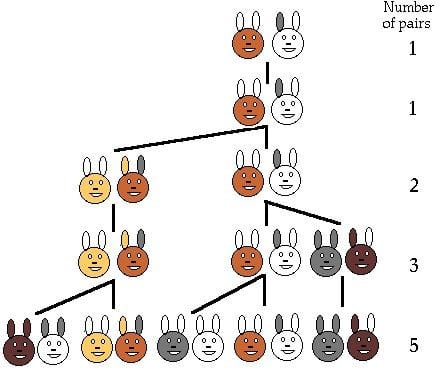# PROBLEM LINK: Contest Page | CodeChef

Practice

Author: Setter’s name

Tester: Tester’s name

Editorialist: Editorialist’s name

DIFFICULTY : BEGINNER

Nill

# PROBLEM:

In a farm, there’s a pair of rabbits. Each month each pair gives birth to a new pair which become reproductive from the second month. How many pairs of rabbits would be there in the farm by the end of each month until the nth month?# QUICK EXPLANATION:

Print the fibonacci series up till the nth number which is given by the user.

# EXPLANATION:

The Fibonacci sequence is a set of numbers that starts with a one or a zero, followed by a one, and proceeds based on the rule that each number is equal to the sum of the preceding two numbers. We first need to input the nth number upto which the sequence is to be printed. After reading the value print the value 1 and then enter a loop that will go on printing the values after 1 up till the nth number in the sequence.

# SOLUTIONS:

Setter's Solution

++++[-<+++++++++++>]>,[>++++++[-<-------->]>+++++++++[-<<<[->+>+<<]>>[-<<+

]>]<<[-<+>]]<<+++++.-----.+++++.----->–>+>+<<[-<.>>>[->+>+<<]<[->>>+<<<

]>>[-<<+>>]>[->+<<<+>>]>[>>>>++++++++++<<<<[->+>>+>-[<-]<[->>+<<<<[->>>+<<<

]>]<<]>+[-<+>]>>>[-]>[-<<<<+>>>>]<<<<]<[>++++++[<++++++++>-]<-.[-]<]<<<<]

Tester's Solution

Same Person

Editorialist's Solution

Same Person